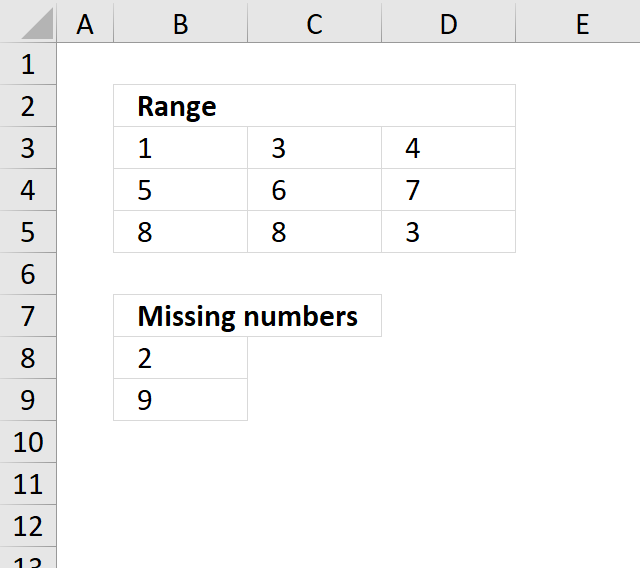Author: Oscar Cronquist Article last updated on December 03, 2018Question: How do I find missing numbers between 1-9 in a range?

1 3 4
5 6 7
8 8 3

Array formula in cell B8:

=SMALL(IF(COUNTIF(\$B\$3:\$D\$5, ROW(\$1:\$9))=0, ROW(\$1:\$9), ""), ROWS(B8:\$B\$8))

If you have numbers between 5 and 32, change ROW(\$1:\$9) to ROW(\$5:\$32)

The formula won´t work if you have numbers above 1048576 (excel 2007). See this post: Identify missing values in a column using excel formula

How to create an array formula

1. Copy above array formula
2. Select cell B8
3. Click in formula bar
4. Paste array formula in formula bar
5. Press and hold Ctrl + Shift
6. Press Enter

Explaining formula in cell B8

Step 1 - Check if numbers in range equal numbers in list

The COUNTIF function counts values based on a condition, in this case, multiple conditions.  The first argument is the cell range containing the numbers, the second argument is a given list of numbers.

The ROW function returns the row number of a cell, in this case a cell range. The ROW function then returns an array of row numbers of all cells in the cell range.

COUNTIF(\$B\$3:\$D\$5, ROW(\$1:\$9)

becomes

COUNTIF({1,3,4; 5,6,7; 8,8,3},{1; 2; 3; 4; 5; 6; 7; 8; 9})

and returns

{1;0;2;1;1;1;1;2;0}.

Step 2 - Which numbers are missing?

A 0 (zero) indicates a number is missing, compare the value with 0 (zero) and we get either TRUE or FALSE.

COUNTIF(\$B\$3:\$D\$5,ROW(\$1:\$9))=0

becomes

{1;0;2;1;1;1;1;2;0}=0

and returns

{FALSE; TRUE; FALSE; FALSE; FALSE; FALSE; FALSE; FALSE; TRUE}.

Step 3 - Replace TRUE with corresponding number

IF(COUNTIF(\$B\$3:\$D\$5, ROW(\$1:\$9))=0, ROW(\$1:\$9), "")

becomes

IF({FALSE; TRUE; FALSE; FALSE; FALSE; FALSE; FALSE; FALSE; TRUE}, ROW(\$1:\$9), "")

becomes

IF({FALSE; TRUE; FALSE; FALSE; FALSE; FALSE; FALSE; FALSE; TRUE}, {1; 2; 3; 4; 5; 6; 7; 8; 9}, "")

and returns

{"";2;"";"";"";"";"";"";9}.

Step 4 - Extract a new number in a cell each

The SMALL function extracts the k-th smallest number from a cell range or array. SMALL( cell_ref, k)

The second argument contains the ROWS function and an expanding cell range. When you copy the cell and paste to cells below the cell reference grows. This makes sure that a new numb er is extracted in each cell.

SMALL(IF(COUNTIF(\$B\$3:\$D\$5, ROW(\$1:\$9))=0, ROW(\$1:\$9), ""), ROWS(B8:\$B\$8)

becomes

SMALL({"";2;"";"";"";"";"";"";9}, ROWS(B8:\$B\$8)

becomes

SMALL({"";2;"";"";"";"";"";"";9}, 1)

and returns 2 in cell B8.